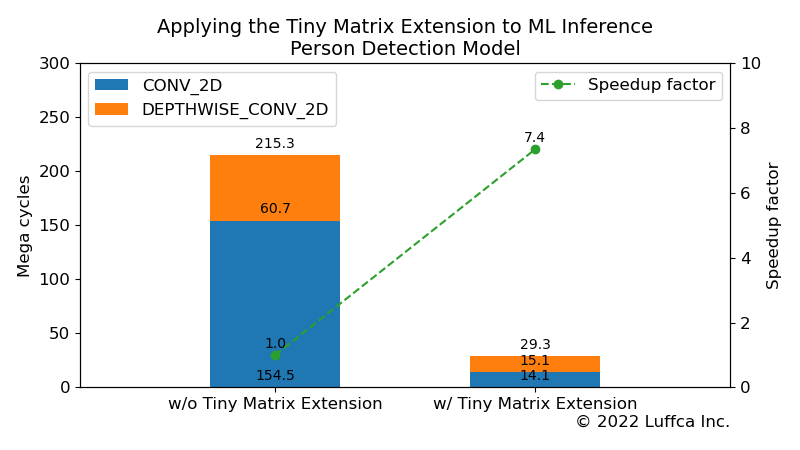# Applying the Tiny Matrix Extension to ML InferenceWe have accelerated machine learning (ML) model inference using a processor that accelerates matrix multiplication at low resource cost. Specifically, inference of the Person Detection model of TensorFlow Lite for Microcontrollers (TFLite Micro) is 7.4 times faster using a RISC-V processor with the Tiny Matrix Extension.

See related articles here.

## Tiny Matrix Extension

Tiny Matrix Extension introduced in the related article Tiny Matrix Extension using RISC-V Custom Instructions is a custom extension that uses RISC-V custom instructions to speed up matrix multiplication at low resource cost.

## Person Detection Model

TFLite Micro’s Person Detection model is an ML model introduced in the related article Building an ML Processor using CFU Playground (Part 1). This model has a total of 31 layers, 14 layers each for `CONV_2D` and `DEPTHWISE_CONV_2D`, and 1 layer each for `AVERAGE_POOL_2D`, `RESHAPE`, and `SOFTMAX`.

In Google’s CFU Playground and the related article’s in-house project, the total number of cycles is reduced to 86M and 38.4M, respectively.

## Applying the Tiny Matrix Extension to Person Detection ModelResult of golden tests for Person Detection model

In applying the Tiny Matrix Extension to the ML model of TFLite Micro, we changed the gateware for the FPGA board Arty A7-35T corresponding to the `input_offset` in the following formula.

```acc += (input_val + input_offset) * filter_val
```

As a result, as shown in the featured image and the table below, the total number of cycles of the Person Detection model decreased from 215.3M to 29.3M, achieving a 7.4 times speedup.

It is 2.9 times faster than Google’s CFU Playground 86M, and 1.3 times faster than the related article’s in-house project 38.4M.

Person Detection
Model
Cycles Speedup
factor
w/o Tiny Matrix
Extension
w/ Tiny Matrix
Extension
`CONV_2D` 154.5M 14.1M 10.9
```DEPTHWISE_ CONV_2D``` 60.7M 15.1M 4.0
Total 215.3M 29.3M 7.4

## Summary

We get a 7.4x speedup in TFLite Micro’s Person Detection model inference using a RISC-V processor with the Tiny Matrix Extension that accelerates matrix multiplication at a low resource cost.

Previous article

Next article